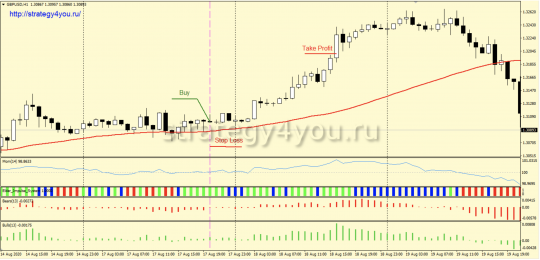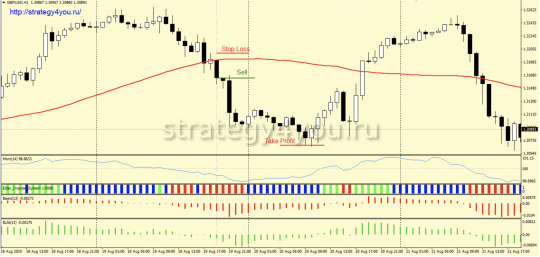# +2950 points — Forex strategy «ZOO» for GBPUSD

Zoo Forex Trading Strategy has a nice set of filters. Signals are obtained quite often, but it is the filters that filter out a significant part of them. Trend signals, of course, are more promising, but reversal signals very often work out well.

Statistics:

From August 2019 to August 2020, the trading strategy showed a positive result of +2950 points. The maximum drawdown was 245 points.

• Time interval — H1.
• Currency pair — GBP/USD.

Indicators:

• Simple moving average with a period of 48 — SMA48.
• Momentum indicator with a period of 14 and with an added level of 100.
• Elder Impulse System indicator.
• Bears Power indicator with a period of 13.
• Bulls Power indicator with a period of 13.

## Conditions for purchases using the ZOO strategy:1) The next candle is closed above the moving average (the price is above the moving average).

2) The Momentum indicator either crosses its zero mark upwards, or bounces upwards from it after touching. One crossing / rebound — one signal.

3) The Elder Impulse System indicator is green.

4) The scale of the Bears Power indicator is growing.

5) The scale of the Bulls Power indicator is growing.

6) The order in which the signals are received does not matter. The main thing is that all the signals in the end be valid on one candle.

7) As soon as all the signals are received, a buy deal is concluded.

8) Stop loss is 35 points.

9) After passing 30 points in the positive zone, the transaction is transferred to breakeven.

10) Take profit is 98 pips.

11) If after the entry a reverse signal is received, and the price is in the positive zone, the transaction is transferred to breakeven. If the price is in the negative zone, then the transaction is closed at the market price.

12) New transactions in the same direction can only be considered if the previous transaction has been moved to breakeven.

## Sales conditions:1) The next candle for the Pound-Dollar is closed below the SMA48 average (the price is below the moving average).

2) The Momentum indicator crosses its «0» mark from top to bottom, or bounces down from it after touching. But you need to remember that 1 intersection / rebound — 1 signal.

3) The Elder Impulse System indicator is red.

4) The Bears Power gauge is dropping.

5) The Bulls Power bar is dropping.

6) The order in which the signals are received does not matter at all. The main thing is that all signals in the end be valid on the 1st candle.

7) As soon as all the signals have been received, a sell deal is concluded.

8 ) Order for deposit insurance — stop loss is equal to 35 points.

9) After the price passes +30 points in the «+» zone, the transaction is transferred to breakeven.

10) We fix the deal at take profit = 98 points.

11) If a reverse signal is received after entering the trade, and the price is in the «+» zone, the trade is transferred to the breakeven point. If the price is in the «-» zone, then the deal is closed at the current market price.

12) New transactions in the same direction (sell) can only be considered if the previous transaction has already been moved to breakeven.

### Video version of the ZOO forex strategy:

• Recommended to watch with English subtitles!

Original